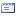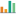### The development of Newton’s method by enhancing the starting point

Ismi Ratin Nabiyah(1), Opim Salim(2), Tulus Tulus(3),
(1) Universitas Sumatera Utara, Medan, Indonesia
(2) Universitas Sumatera Utara, Medan, Indonesia
(3) Universitas Sumatera Utara, Medan, IndonesiaCorresponding Author

#### Abstract

In general, nonlinear problems cannot be solved analytically. A special theory or method is needed to simplify calculations. Many problems that are too complex, an exact solution is needed to support numerical solution. There are many numerical methods that can be used to solve nonlinear problems, including the Bisection, Secant and Newton methods, also known as the Newton-Raphson method. However, these methods cannot be used for large-scale of nonlinear programming problems; for example the Newton-Raphson method which does not always converge if it takes the wrong initial value. The Newton-Raphson method is widely used to find approximations to the roots of real functions. However, the Newton-Raphson method does not always converge if it takes the wrong initial value. Therefore, it is necessary to develop the Newton-Raphson method without using other methods in order to have a higher convergence. This research is a literature study compiled based on literature references with the initial step of understanding problems that appear from the use of Newton's method, it is base on the problem of divergence or oscillation. Newtonian method was developed without modification of other methods, but took two starting points. Then prove the super-quadratic convergence of the proposed method by extending the Taylor expansion and giving or assuming the error rate. After that, the stability test of the proposed model is carried out and provides an example of the application by solving the root search using Newton's method and the proposed method can be seen as a comparison.

#### Keywords

development; newton’s method; newton-raphson; starting point

#### Article DOI

DOI: https://doi.org/10.33122/ijtmer.v6i1.205

#### Article MetricsAbstract views : 30

Pages:

#### References

Azizi, A. Abdi, S. 2015. A new idea for constructing Halley’s method.Internationalv Journal of Applied Mathematics. Vol. 28, 107-110.

Behl, R dan Kanwar, V. 2013. Variants of Chebyshev’s Method with Optimal Order of Convergence. Tamsui Oxford Journal of International and Mathematical Sciences. Vol. 29, 39-53.

Chen, J. Vuik, C. 2016. Globalization tecjnique for projected Newton-Krylov method. International Journal for National Method’s in Engineering.

Chun, C. 2007. Some improvements of Jarratt’s method with sixth-order convergence. Appl. Math.Comput. Vol. 190, 1432-1437.

Traub, J.F. 1982. Iterative methods for the solution of equations, Englewood: Prentice-Hall.

Sharma, J.R, Guha, R.K. 2007. A family of modiefied Ostrowski methods with accelerated sixth order convergence. Appl. Math. Comput. Vol.190, 111-115.

Kanwar, V. dan Tomar, S.K. 2009. Exponentially fitted variants of Newton’s methods with quadratic and cubic convergence. Journal of Computer Mathematics. Vol. 86, 1603-1611.

Kho, J. 2014. Steffensen-Newton method with third order convergence to solve nonlinear equation. Journal of Applied Mathematics. Vol. 140, 519-522.

Kreyszig E. 1998. Advanced Engineering Mathematics 8th edition. New York: John Wiley.

Sanchez, M.G. dan Barrero, J.L.D. 2011. A Technique to composite a modiefied Newton’s method for solving nonlinear Equations. Cornell University Library.

Weerakoon, S. dan Fernando. 2000. A variant of Newton’s methode with accelerated third order convergence. Applied Mathematics Letters. Vol.13, 87-93.

### Refbacks

• There are currently no refbacks.

Copyright (c) 2023 Ismi Ratin Nabiyah, Opim Salim, Tulus Tulus

International Journal of Trends in Mathematics Education Research (IJTMER)

E-ISSN 2621-8488